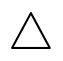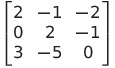Select Page

# Relations and Functions MCQ CBSE Maths 12 Science Solutions in English

Relations and Functions MCQ CBSE Maths 12 Science Solutions in English to enable students to get Solutions in a narrative video format for the specific question.

Expert Teacher provides Relations and Functions MCQ CBSE Maths 12 Science Solutions through Video Solutions in English language. This video solution will be useful for students to understand how to write an answer in exam in order to score more marks. This teacher uses a narrative style for a question from Relations and Functions not only to explain the proper method of answering question, but deriving right answer too.

Please find the question below and view the Solution in a narrative video format.

Question:

Solution Video in English:

You can select video Solutions from other languages also. Please check Solutions in ( Hindi )

## Similar Questions from CBSE, 12th Science, Maths, Relations and Functions

Question 1 : Let A = {1, 2, 3, 4} and let R = {(2, 2), (3, 3), (4, 4), (1, 2)} be a relation on A. Then, R is, (View Answer Video)

Question 2 : Let R be the relation in the set N given by :
R = {(a, b) : a = b - 2, b > 6}.

Question 3 : A functiondefined asis, (View Answer Video)

Question 4 : Let * be the binary operation on N given by a * b = LCM of a and b. Find the identity of * in N? (View Answer Video)

Question 5 : Functionsare defined respectively, by, find. (View Answer Video)

### Inverse Trigonometric Functions

Question 1 :is equal to : (View Answer Video)

Question 2 : Evaluate :(View Answer Video)

Question 3 : Evaluate:(View Answer Video)

Question 4 :then find the value of x. (View Answer Video)

Question 5 : Using principal values, write the value of(View Answer Video)

### Determinant

Question 1 : If x, y, z are non-zero real numbers, then the inverse of matrixis, (View Answer Video)

Question 3 : If=andis Cofactor of, then the value ofis given by (View Answer Video)

Question 4 : Find the equation of the line joining (3, 1) and (9, 3) using determinants. (View Answer Video)

Question 5 :  Evaluate the determinants:. (View Answer Video)

### Vector Algebra

Question 1 : Find the angle between two vectors a and b with magnitudesand 2 respectively, having(View Answer Video)

Question 2 : Find the vector quantities :
(i)     Work                     (ii)      Force
Question 3 :  Write a vector in the direction of the vectorthat has magnitude 9 units.   (View Answer Video)
(i) 10 kg          (ii) 2 m north-west          (iii)(iv) 40 W         (v)(vi)(View Answer Video)